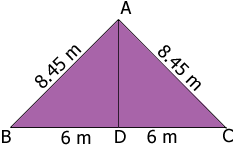SEARCH HOMEMath Central Quandaries & QueriesQuestion from Brian: the base of my triangle is 12 metres and the two sides are 8.45 metres can you help me find the height of the triangleHi Brian,

In my diagram D is the midpoint of the base $BC.$The angle $ADC$ is a right angle. Do you see why? Thus $|AD|$ is the height of the triangle. Pythagoras tell you the rest.

PennyMath Central is supported by the University of Regina and The Pacific Institute for the Mathematical Sciences.